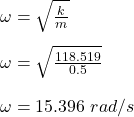## A 0.5 kg mass on a spring undergoes simple harmonic motion with a total mechanical energy of 12 J. If the oscillation amplitude is 0.45 m, w

Question

A 0.5 kg mass on a spring undergoes simple harmonic motion with a total mechanical energy of 12 J. If the oscillation amplitude is 0.45 m, what is the frequency of the oscillation?

in progress 0
5 months 2021-09-05T13:53:28+00:00 1 Answers 0 views 0

## Answers ( )

The frequency of the oscillation is 2.45 Hz.

Explanation:

Given;

mass of the spring, m = 0.5 kg

total mechanical energy of the spring, E = 12 J

Determine the spring constant, k as follows;

E = ¹/₂kA²

kA² = 2E

k = (2E) / (A²)

k = (2 x 12) / (0.45²)

k = 118.519 N/m

Determine the angular frequency, ω;Determine the frequency of the oscillation;

ω = 2πf

f = (ω) / (2π)

f = (15.396) / (2π)

f = 2.45 Hz

Therefore, the frequency of the oscillation is 2.45 Hz.